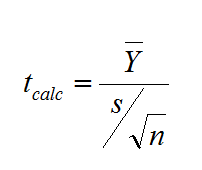# Tag Archives | one sample t-Test## One Sample t Test with SigmaXL

What is a One Sample t Test? A One sample t test is a hypothesis test to study whether there is a statistically significant difference between a population mean and a specified value. Where: What is a t-Test? In statistics, a t-test is a hypothesis test in which the test statistic follows a Student’s t […]